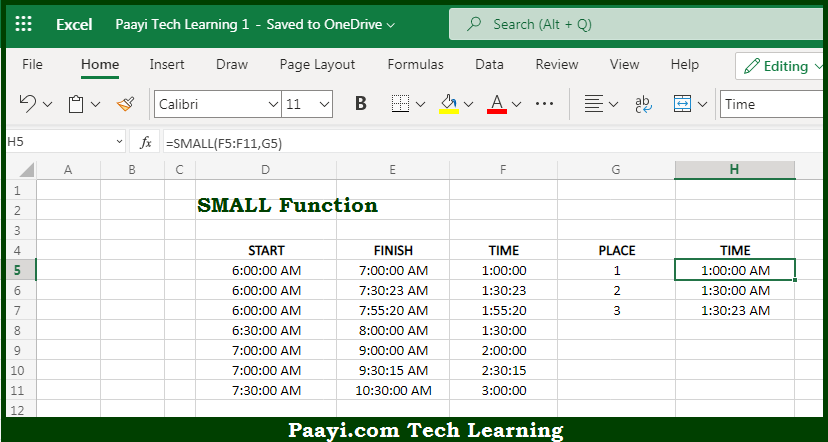# Learn How to Use Microsoft Excel SMALL Function

Written by | 0 Comments | 593 Views

In this article, you will learn how to use the Microsoft Excel SMALL function and its prime function in Microsoft Excel. You will also get to know the Microsoft Excel SMALL function return value and syntax with the help of some examples.

Microsoft Excel SMALL Function

The main purpose of the Microsoft Excel SMALL function is to get the nth smallest value. That implies, with the help of the SMALL function you can able to return the numeric values based on their position in a list ranked by the value. It can be understood as, it can retrieve "nth smallest" values like - 1st smallest value, 2nd smallest value, 3rd smallest value, and so on. So, with the help of the SMALL function, you can able to get the nth smallest value.

Return Value of SMALL Function

The return value will be the nth smallest value in the array.

Syntax of SMALL Function

=SMALL(array, n)

Where the arguments:

• array: This is the range of cells from which you want to extract the smallest values.
• n: This is the integer that specifies the position from the smallest value, i.e. the nth position.

## How to Use Microsoft Excel SMALL Function?So we know that Microsoft Excel SMALL function you can able to get the nth smallest value. That implies, with the help of the SMALL function you can able to return the numeric values based on their position in a list ranked by the value. It can be understood as, it can retrieve "nth smallest" values like - 1st smallest value, 2nd smallest value, 3rd smallest value, and so on. So, with the help of the SMALL function, you can able to get the nth smallest value.